### Home > CALC > Chapter 4 > Lesson 4.2.1 > Problem4-45

4-45.
1. Use a Riemann sum with 20 rectangles to approximate the following integrals. Then use the numerical integration feature of your graphing calculator to check your answer. Homework Help ✎

1.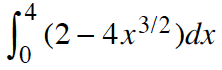2.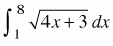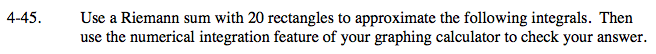General form of left-endpoint Riemann sum:

$\sum_{i=0}^{n-1}\Delta xf(a+\Delta xi)$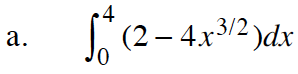$\text{Riemann approximation: }\approx \sum_{i\rightarrow 0}^{19}\frac{1}{5}f\left ( 0+\frac{i}{5} \right )=\sum_{i\rightarrow 0}^{19}\frac{1}{5}\left ( 2-4\left ( \frac{i}{5} \right )^{\frac{3}{2}} \right )=-40.04$

$\text{Your calculator should reveal that }\int_{0}^{4}\left ( 2-4x^{\frac{3}{2}} \right )=-43.2.$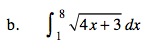Refer to part (a).